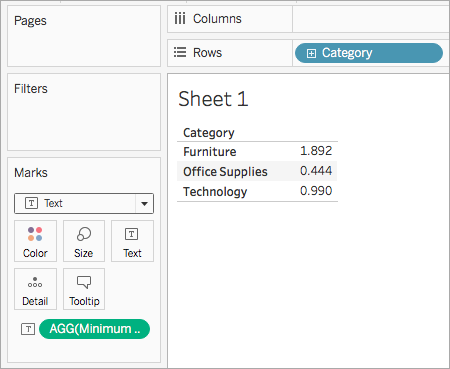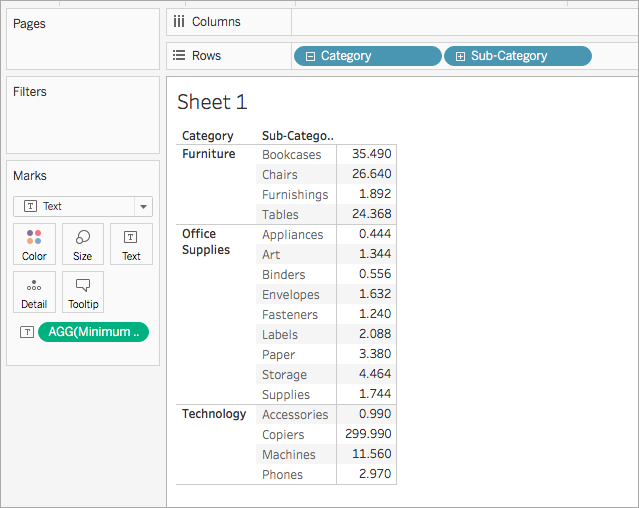# 数字函数

## 为何使用数字函数

`ABS[Budget Variance]`

## Tableau 中的可用数字函数列表

#### 描述

ABS

`ABS(number)`

`ABS(-7) = 7`
`ABS([Budget Variance])`

ACOS

`ACOS(number)`

```ACOS(-1) = 3.14159265358979```

ASIN

`ASIN(number)`

`ASIN(1) = 1.5707963267949`

ATAN

`ATAN(number)`

```ATAN(180) = 1.5652408283942```

ATAN2

`ATAN2(y number, x number)`

`ATAN2(2, 1) = 1.10714871779409`

CEILING

`CEILING(number)`

`CEILING(3.1415) = 4`

#### 按数据源的可用性：

 数据源 Support Microsoft Access 不支持 Microsoft Excel 支持 文本文件 支持 统计文件 支持 Tableau Server 支持 Actian Vector 不支持 Amazon Aurora for MySQL 不支持 Amazon EMR Hadoop Hive 支持 Amazon Redshift 支持 Aster Database 不支持 Cloudera Hadoop 支持 DataStax Enterprise 支持 EXASOL 不支持 Firebird 不支持 Google Analytics（分析） 支持 Google BigQuery 支持 Google Cloud SQL 不支持 Google Sheets 不支持 Hortonworks Hadoop Hive 支持 IBM BigInsights 不支持 IBM DB2 不支持 IBM PDA (Netezza) 不支持 MapR Hadoop Hive 支持 MarkLogic 不支持 Microsoft Analysis Services 不支持 Microsoft PowerPivot 不支持 Microsoft SQL Server 支持 MySQL 不支持 Oracle 不支持 Oracle Essbase 不支持 Actian Matrix (ParAccel) 不支持 Pivotal Greenplum 不支持 PostgreSQL 不支持 Progress OpenEdge 不支持 Salesforce 支持 SAP HANA 不支持 SAP Sybase ASE 不支持 SAP Sybase IQ 不支持 Spark SQL 支持 Splunk 不支持 Teradata 不支持 Teradata OLAP Connector 不支持 Vertica 不支持

COS

`COS(number)`

`COS(PI( ) /4) = 0.707106781186548`

COT

`COT(number)`

`COT(PI( ) /4) = 1`

DEGREES

`DEGREES(number)`

`DEGREES(PI( )/4) = 45.0`

DIV

DIV(整数 1, 整数 2)

`DIV(11,2) = 5`

EXP

`EXP(number)`

`EXP(2) = 7.389`
```EXP(-[Growth Rate]*[Time])```

FLOOR

`FLOOR(number)`

`FLOOR(3.1415) = 3`

#### 按数据源的可用性：

 数据源 Support Microsoft Access 不支持 Microsoft Excel 支持 文本文件 支持 统计文件 支持 Tableau Server 支持 Actian Vector 不支持 Amazon Aurora for MySQL 不支持 Amazon EMR Hadoop Hive 支持 Amazon Redshift 不支持 Aster Database 不支持 Cloudera Hadoop 支持 DataStax Enterprise 支持 EXASOL 不支持 Firebird 不支持 Google Analytics（分析） 支持 Google BigQuery 支持 Google Cloud SQL 不支持 Hortonworks Hadoop Hive 支持 IBM BigInsights 不支持 IBM DB2 不支持 IBM Netezza 不支持 MapR Hadoop Hive 支持 MarkLogic 不支持 Microsoft Analysis Services 不支持 Microsoft PowerPivot 不支持 Microsoft SQL Server 支持 MySQL 不支持 Oracle 不支持 Oracle Essbase 不支持 ParAccel 不支持 Pivotal Greenplum 不支持 PostgreSQL 不支持 Progress OpenEdge 不支持 Salesforce 支持 SAP HANA 不支持 SAP Sybase ASE 不支持 SAP Sybase IQ 不支持 Spark SQL 支持 Splunk 不支持 Teradata 不支持 Teradata OLAP Connector 不支持 Vertica 不支持

HEXBINX

`HEXBINX(number, number)`

HEXBINX 和 HEXBINY 是用于六边形数据桶的分桶和标绘函数。六边形数据桶是对 x/y 平面（例如地图）中的数据进行可视化的有效而简洁的选项。由于数据桶是六边形的，因此每个数据桶都非常近似于一个圆，并最大程度地减少了从数据点到数据桶中心的距离变化。这使得聚类分析更加准确并且能提供有用的信息。

`HEXBINX([Longitude], [Latitude])`

HEXBINY

`HEXBINY(number, number)`

`HEXBINY([Longitude], [Latitude])`

LN

`LN(number)`

LOG

`LOG(number [, base])`

MAX

`MAX(number, number)`

`MAX(4,7)`
`MAX(Sales,Profit)`
```MAX([First Name],[Last Name])```

MIN

`MIN(number, number)`

`MIN(4,7)`
`MIN(Sales,Profit)`
```MIN([First Name],[Last Name])```

PI

`PI( )`

POWER

`POWER(number, power)`

```POWER(5,2) = 52 = 25```
`POWER(Temperature, 2)`

```5^2 = POWER(5,2) = 25```

`RADIANS(number)`

`RADIANS(180) = 3.14159`

ROUND

`ROUND(number, [decimals])`

`ROUND(Sales)`

SIGN

`SIGN(number)`

```SIGN(AVG(Profit)) = -1```

SIN

`SIN(number)`

```SIN(0) = 1.0```
`SIN(PI( )/4) = 0.707106781186548`

SQRT

`SQRT(number)`

`SQRT(25) = 5`

SQUARE

`SQUARE(number)`

`SQUARE(5) = 25`

TAN

`TAN(number)`

```TAN(PI ( )/4) = 1.0```

ZN

`ZN(expression)`

`ZN([Profit]) = [Profit]`

## 创建数字计算

1. 在 Tableau Desktop 中，连接到 Tableau 附带的示例 - 超市已保存数据源。

2. 导航到工作表，并选择“分析”>“创建计算字段”

3. 在打开的计算编辑器中，执行以下操作：

• 将计算字段命名为“Minimum Sales transaction”（最低销售额交易）

• 输入以下公式：

`MIN(Sales)`

• 完成后，单击“确定”## 另请参见

Tableau 中的函数

Tableau 函数（按字母顺序）

Tableau 函数（按类别）